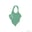Related Tags

js
function
javascript
twcompetition
communitycreator

# How to use the Abs() method in JavaScriptLateefat Salami

## What is the abs() method?

From mathematics, we know that the absolute value of a number is the distance from zero that a number is on the number line without considering its direction.

The abs() method works the same way in JavaScript. It returns the absolute value of the parameter (number) that is passed into it.

## Syntax

Math.abs(parameter);

Note: The Math object allows the use of mathematical functions. So, the abs() method is called using this object.

Parameter

x

Details

Usually a number

## Example 1

The following is an example of the abs() method with numbers:

  /* educative.io
abs() with numbers*/

console.log("The abs() method with Numbers \n");
console.log (Math.abs(5));
console.log (Math.abs(-5));
console.log (Math.abs(4*-5)); //same as |-20|
console.log (Math.abs(5-8));  //same as |-3|

From the example above, we can see that the code outputs positive (absolute) values in all cases.

## Example 2

The following is an example of the abs() method with other parameters:

/* educative.io
abs() with other parameters*/

console.log("The abs() method with other parameters \n");

// when no(null) parameter is passed
console.log (Math.abs(null));
console.log (Math.abs(""));
console.log (Math.abs([]));

// for lists and Strings
console.log (Math.abs("Welcome to Edpresso!"));
console.log (Math.abs([7, 5, 3, 1]));

In this example, the return value for the null parameter is 0. The code outputs Nan when a string or list is passed into it.

RELATED TAGS

js
function
javascript
twcompetition
communitycreator

CONTRIBUTORLateefat Salami
RELATED COURSES

View all Courses

Keep Exploring

Learn in-demand tech skills in half the time